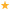### Seating Chart

For iPad users, click on a seat once to view seat info, click twice to select the seat.

Show schedules:

\$75.00

\$65.00

\$55.00

\$50.00

\$45.00In Cart
0 selected

A
A
B
B
C
C
D
D
E
E
F
F
G
G
H
H
J
J
K
K
A
A
B
B
C
C
D
D
E
E
F
F
G
G
H
H
J
J
K
K
A
A
B
B
C
C
D
D
E
E
F
F
G
G
H
H
J
J
K
K

## Mezzanine

L
L
M
M
N
N
O
O
P
P
Q
Q
R
R
S
S
T
T
U
U
V
V
Q
Q
R
R
S
S
T
T
U
U
V
V
L
L
M
M
N
N
O
O
P
P
Q
Q
R
R
S
S
T
T
U
U
V
V
Q
Q
R
R
S
S
T
T
U
U
L
L
M
M
N
N
O
O
P
P
Q
Q
R
R
S
S
T
T
U
U
V
V
Q
Q
R
R
S
S
T
T
U
U
V
V
L
L
M
M
N
N
O
O
P
P
Q
Q
R
R
S
S
T
T
U
U
V
V

\$75.00

\$65.00

\$55.00

\$50.00

\$45.00In Cart